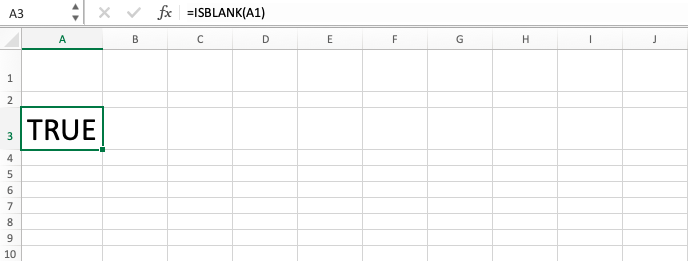ISBLANK Excel Formula - Compute Expert

# ISBLANK Excel Formula

In this tutorial, it will be explained about all the things related to the ISBLANK excel formula. ISBLANK function in excel is useful to check whether a cell is empty or not. It gives you TRUE logical value if yes and FALSE if not.

## Why do We Need to Learn ISBLANK Function in Excel?

In the data processing on a spreadsheet, sometimes we want to check whether there is an empty cell in the range where we put the data or not. This is needed so we can make sure that there is no lack of data that can negatively impact the further processing process that you need.

Maybe we want to do this checking process manually. But, this will be much harder to do if there are too many cells in a range that we must check.

As an example of the need for a job, imagine we want to do data processing to the daily sales numbers of all products in a company. Before doing further analysis of all the numbers, maybe it will be better if we can check every cell in the range where all the sales numbers are in to ensure that the sales data that want to be processed are already complete. If we need to analyze the company products’ sales in months or even years, then there will be much data. It will be troublesome if we need to check each of the cells whether they are empty or not. However, the dilemma is the checking process must be done so there will be no error or mistake from the analysis process later.

To help you check, you can use a fast way by utilizing the ISBLANK excel formula. ISBLANK function in excel will give TRUE if a cell is checked empty and FALSE if not. It will be very useful if you often face the need to check the emptiness of your cell on the spreadsheet just as in the case above.

## What is ISBLANK Excel Formula?

ISBLANK excel formula is a formula with the usage objective to check whether a cell is empty or not and get the logic value of TRUE or FALSE depending on your checking result. ISBLANK function in excel can also be used if you want to give an alternative value to your empty cell through its combination with the IF formula.

This formula is one of the IS formulas in the spreadsheet besides others like ISNA or ISERROR that can be used to do the checking process for different needs.

Generally, the input of this function can be explained briefly as follows:

=ISBLANK(value)

Note:
value = the cell that wants to be checked whether it is empty or not

## How to Use ISBLANK Function in Excel?

The following will explain how to write the ISBLANK excel formula. ISBLANK function in excel only needs the input of a cell coordinate that you want to check the emptiness of.

## Using ISBLANK Excel Formula

1. Type equal sign ( = ) in the cell where you want to put the result in2. Type ISBLANK (can be with large and small letters) and open bracket sign after =3. Input the cell coordinate to be checked after open bracket sign4. Input close bracket sign5. Press Enter
6. The process is done!## Exercise

After you have understood how to write ISBLANK function in excel from the tutorial above, now is the time you do the exercise to sharpen your understanding!

### Instructions

Answer each question in the gray-colored places in the appropriate number row!
1. Is the cell in your left empty? Answer with TRUE if yes and FALSE for no!
3. Is the cell in your left empty? Answer with YES if yes and NO for no! Use IF also to help you in answering this question (Probably you are confused with your result from this question? Look at the explanation in the additional note part of this tutorial)

If a formula results in empty value, then the ISBLANK function in excel will still evaluate the cell where the formula is in as not empty because there is the formula in there. ISBLANK excel formula only gives TRUE if there is absolutely nothing in your cell.

Get updated excel info from Compute Expert by registering your email. It's free!